# RBSE Solutions For Class 12 Physics Chapter 9: Electromagnetic Induction | Textbook Important Questions & Answers

The answers are provided for all the questions of Chapter 9 Physics of RBSE Class 12. Students can go through these questions to understand the concepts better and score well in the board examination and entrance examinations for various professional courses. These solutions are provided by the team of experts to provide the best and accurate solutions to the questions. Do check BYJU’S RBSE Class 12 solutions page to get the answers for textbook questions of all subjects.

## Multiple Choice Questions

Q1: If a conducting rod moves with a constant velocity v in a magnetic field, emf is induced between both its ends if

1. V and B are parallel
2. V is perpendicular to B
3. V and B are in opposite directions
4. All the above

Q2: The unit of the ratio of magnetic flux to resistance is equal to the unit of which quantity

1. Charge
2. Potential difference
3. Current
4. Magnetic field

Q3: Induced emf of electromagnetic induction depends upon

1. Resistance on conductor
2. The value of magnetic field
3. The direction of conductor w.r.t the magnetic field
4. Rate of change of flux linked

Q4: When a bar magnet is entered into a coil, the induced emf in the coil does not depend upon

1. Speed of magnet
2. Number of turns in coil
3. Magnetic moment of magnet
4. Resistance of wire in coil

Q5: A coil of copper wire is placed parallel to a uniform magnetic field, then the induced emf will be

1. Infinite
2. Zero
3. Equal to magnetic field
4. Area of coil

## Very short Questions

Q1: If the current in inductance is doubled then how many times does the stored energy increase?

Answer: From the relation, W = (½)LI2, the stored energy increases by 4 times

here I is the current and W is the energy stored

Q2: Why does a spark occur when an electric circuit is suddenly broken?

Answer: The spark occurs due to the large amount of induced Electromotive force.

Q3: How can the mutual inductance between the coils be increased?

Answer: The mutual inductance can be increased by increasing the number of turns in a coil.

Q4: On doubling the area of cross-section while keeping the same number of turns in a coil, what will be the value of self-inductance?

Answer: The self-inductance of the coil is proportional to the area. If the area is doubled then self-inductance value also will be doubled.

Q5: How can we reduce the effect of Eddy currents in the core of a transformer?

Answer: Eddy current can be reduced by using a soft iron core.

Q6: A metallic and a non-metallic coin are dropped from the same height towards the earth surface. Which coin reaches earlier on earth and why?

Answer: The non-metallic coin reaches earlier on the surface of the earth. There will be an effect of eddy current on the metallic coin.

Q7: Why self-induction is called electrical inertia?

Answer: Self-induction is called electrical inertia due to the opposing nature of growth or decay of current in the coil.

Q8: On what factors does the self-inductance of the solenoid depend?

Answer: The equation of self-inductance of the solenoid is given by

L=μ0N2A/l

Here μ0 is the permeability of free space

N is the number of turns

A is the area of each turn of solenoid

L is the length of the solenoid

Q9: In a wire, current flows at high voltage. As current is switched on the wire why the bird sitting on wire fly?

Answer: They fly due to the effect of induced current.

Q10: Write down dimensions of L/R here L is self-inductance and R is resistance.

Answer: The dimensional formula is given by [M0L0T1A0]

Q11: When a rectangular loop moves in a uniform magnetic field with constant speed then what is the magnitude of induced emf?

Answer: The magnetic field induced will be zero due to the change in magnetic flux.

Q12: In what way, two coils are wounded so that induced emf is maximum?

Answer: The magnetic field produced in the coil is in the same direction.

Q13: If a coil or rectangular loop rotates in a magnetic field what factors affect the induced emf in it?

Answer: The emf induced is given by

Є=Є0sinωt =NABωsinωt

N is the number of turns

A is the area of cross-section

B is the magnetic field

ω is the angular velocity

Q14: A straight and long wire is dropped freely gravitational field keeping in the north-south direction, why emf is induced in the wire?

Answer: There will not be any change in the magnetic flux.

Q15: How eddy currents are used to make the galvanometer dead beat?

Answer: Eddy currents are produced in the soft iron core which opposes the motion of the pointer.

Q1: What do you mean by electromagnetic induction? Write down Faraday’s Law for electromagnetic induction and magnitude of induced emf?

Electromagnetic Induction is a current produced because of voltage production (electromotive force) due to a changing magnetic field.

Faraday’s Law for electromagnetic induction: Faraday gave two laws based on the results of his experiments.

Faraday’s First Law: Whenever the amount of magnetic flux linked with a closed circuit changes, an emf is induced in it which lasts only till the change in flux is taking place.

Faraday’s Second Law: According to the second law, the magnitude of induced emf is equal to the rate of change in magnetic flux.

The magnitude of induced emf Є = N x dΦ/ dt

Where Є is the induced emf

N is the number of turns

Φ is the magnetic flux

t is the time

Q2. If a coil is removed from the magnetic field (i) rapidly (ii)slowly, then in which situation induced emf and work done is more?

Answer: The induced emf is given by Є = N x dΦ/ dt. If the coil is removed from the magnetic field rapidly the time dt is minimum so the induced emf will be more.

Q3: Write down Len’s Law for electromagnetic induction and explain that Lenz’s law follows the law of energy conservation.

Lenz’s law states that induced electromotive force with different polarities induces a current whose magnetic field opposes the change in magnetic flux through the loop in order to ensure that original flux is maintained through the loop when current flows in it.

Lenz’s law ensures that if the electrical energy gained is 1 unit then the mechanical energy lost will also be equal to 1 unit. Therefore, the law follows conservation of energy.

Q4: Why is there an opposing force experienced while pulling or pushing a metallic plate in a uniform magnetic field?

Answer: When there is a change in magnetic flux, eddy currents are produced in the wire. This causes an opposing force while pulling or pushing a metallic plate in a uniform magnetic field.

Q5: How can we reduce the effect of eddy currents in the core of a transformer?

Answer: The eddy current effect can be reduced in the transformer by increasing the resistance of the core. This can be done by laminating the thin sheets of steel, as the laminations are thin they will have high resistance.

Q6: What is the reason

(i) Resistance wire coils are doubly twisted in resistance boxes

(ii) In Wheatstone Bridge why cell key is pressed first and then galvanometer key?

(i) In the resistance boxes, the coils are doubly twisted in order to nullify the effect of inductance. The magnetic induction caused by both halves of the wire cancel each other and the resistor is left with pure resistance.

(ii) If the galvanometer key is pressed before the cell key the induced key will affect the main current

Q7: Write down Fleming’s right-hand rule for the direction of the induced current?

Fleming’s right-hand rule

“Hold the right-hand forefinger, middle finger and the thumb at right angles to each other. If the forefinger represents the direction of the magnetic field, the thumb points in the direction of motion or applied force, then the middle finger points in the direction of the induced current.”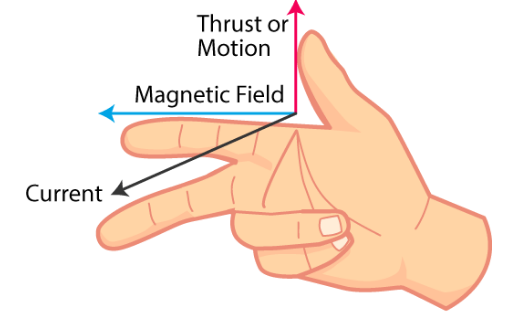Q8: Define mutual inductance and write its unit and dimensions.

When two coils are brought in closer to each other the magnetic field in one of the coils tend to link with the other. The current flowing in one coil further leads to the generation of voltage in the second coil. This property of a coil which affects or changes the current and voltage in a secondary coil is called mutual inductance.

The dimensional formula of mutual inductance is [ML2T-2A-2]. The SI unit is Henry.

Q9: A conducting rod of length L is rotating with uniform angular velocity ω in magnetic field B in such a way that the plane of rotation is perpendicular to the magnetic field then find out the induced emf between its ends?

Consider an infinitesimally small length of rod at a distance x from the centre of the circular path.

The emf induced across this element dє = Bvdx

Here B is the magnetic field, v is the velocity and dx is the element of the rod

If x is the distance from the small element to the centre, then v=ωx

Thus dє = Bωxdx

Integrate the above equation to find the net emf

є = Bωxdx

є =BωL2/2

Q10: Coils A and B are placed perpendicular to each other as shown in fig. If the current in any one coil varies then will the current be induced in the coil? Why?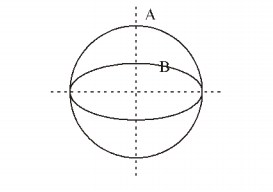Answer: There is no change in magnetic flux in the rotation of the coil. Therefore, there will be no current induced in another coil.

Q11: What are the factors that affect the mutual inductance between two coil. Explain?

1. Effect of the material of the core

The mutual inductance is given by the equation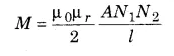From the equation, we understand M∝ μr

The mutual inductance increases when a material with high relative permeability is used.

1. Effect of the number of turns

From the equation, we know M∝N1N2

If the number of turns N1 and N2 are increased then the mutual inductance can be increased

(c) Effect of separation: When the separation between the coil is increased the flux linkage decreases as a result of which mutual inductance decreases.

(d) Effect of coupling: If the self-inductance of the two coils is given by L1 and L2 then their mutual inductance is given by

M=K

Here K is the coupling coefficient

When k is equal to 1 such that all the lines of flux of one coil cuts all of the turns of the second coil, it means that the two coils are tightly coupled together, the resulting mutual inductance will be equal to the geometric mean of the self-inductances of the individual coils.

Q12: Prove that induced charge q = (N/R)(Φ12) when flux associated with a coil changes from

Φ1 to Φ2. Here N is the number of turns in the coil, R is its resistance.

The induced emf in the coil of N turns,

Є = -N(dΦ/dt)

If R is the resistance of the coil then induced current is given by

I =Є/R = (-N/R)(dΦ/dt)

The charge induced in the coil at the time interval dt,

dq = Idt

dq = (-N/R)(dΦ)

If there is a change in flux from Φ1 to Φ2

Then

dq = (-N/R)dΦ

q = (-N/R)(Φ1 – Φ2)

when flux associated with a coil changes from

Φ1 to Φ2. and N is the number of turns in the coil and R is its resistance then

q = (-N/R)(Φ1 – Φ2)

Q13: If the self-inductance of a coil is 1 H, what do you understand by it?

The Self Inductance of the coil L =1 H

Induced emf є = -L(dI/dt)

Since L=1

є = dI/dt

When the self-inductance of the coil is unity then the decay rate of current is equal to the induced emf in the coil.

## Essay Type Questions

Q1: Find out induced emf due to motion of conducting rod in a uniform magnetic field with a constant velocity. How can we find the direction of induced emf?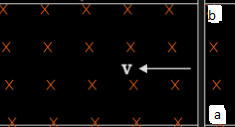Consider a straight conductor as shown in the figure, moving in a uniform field B perpendicular to the plane of the system. Let us consider the rod to move at a constant velocity of v m/sec and the surface to be frictionless. The electrons present in the rod also move with the velocity v in the magnetic field B. The magnetic force on these free electrons is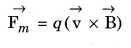Here q is the charge of the electron. For a positive charge, the direction of (v x B) is from b to a. For a negative charge of the electron, the direction of magnetic force is from a to b. Due to drift velocity, the electrons are present in large amounts at end b and lesser at the end a. The end b is negatively charged and the end a is positively charged.

When both the ends of different charges come close then an electrostatic force is created between the ends. This will continue until the force on the electric field is not balanced by the force on electrons due to the magnetic field.

If the electric field developed is E, then the force that acts on an electron of charge q is given by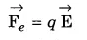In the equilibrium state,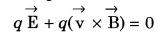The above equation can be written as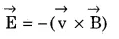Due to the electric field, an induced emf is produced

є = force on unit positive charge x displacement

є = El

є = vBl

If the direction of l is from negative charge end to positive charge end and the conducting rod is moving at an angle θ with the direction of magnetic field lines then the component of v perpendicular to B is given by vsinθ. The induced emf between the ends of the conductor is given by Bvlsinθ. The emf induced will be zero if the conductor moves along the direction of the magnetic field.

Q2: What is self-induction? Explain the experiments of self-induction and calculate the self-inductance for a solenoid.

Self-induction: When there is a change in the current or magnetic flux of the coil, an opposed induced electromotive force is produced. This phenomenon is termed as Self Induction. When the current starts flowing through the coil at some instinct magnetic flux becomes directly proportional to the current passing through the circuit. The relation is given as:

Φ∝I

Φ= LI

Where L is termed as self-inductance of the coil or the coefficient of self-inductance. The self-inductance depends on the cross-sectional area, the permeability of the material or the number of turns in the coil.

The rate of change of magnetic flux in the coil is given as,

є = -(dΦ/dt) = -(dLI/dt)

є = -L(dI/dt)

Self-inductance L = N(Φ/I)

Where

L is the self-inductance

N is the number of turns

Φ is the magnetic flux

I is the current

Experiments of Self Induction

Experiment 1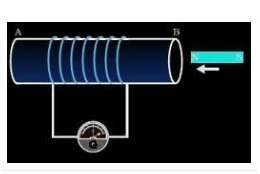In this experiment, Faraday connected a coil to a galvanometer, as shown in the figure above. A bar magnet was pushed towards the coil, such that the north pole is pointing towards the coil. As the bar magnet is shifted, the pointer in the galvanometer gets deflected, thus indicating the presence of current in the coil under consideration. It is observed that when the bar magnet is stationary, the pointer shows no deflection and the motion lasts only till the magnet is in motion. Here, the direction of deflection of the pointer depends upon the direction of motion of the bar magnet. Also, when the south pole of the bar magnet is moved towards or away from the coil, the deflections in the galvanometer are opposite to that observed with the north-pole for similar movements. Apart from this, the deflection of the pointer is larger or smaller depending upon the speed with which it is pulled towards or away from the coil. The same effect is observed when instead of the bar magnet, the coil is moved and the magnet is held stationary. This shows that only the relative motion between the magnet and the coil is responsible for the generation of current in the coil.

Experiment 2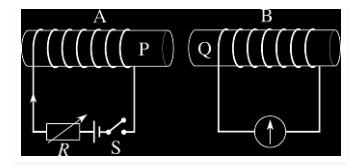In the second experiment, Faraday replaced the bar magnet by a second current-carrying coil that was connected to a battery. Here, the current in the coil due to the connected battery produced a steady magnetic field, which made the system analogous to the previous one. As we move the second coil towards the primary coil, the pointer in the galvanometer undergoes deflection, which indicates the presence of the electric current in the first coil. Similar to the above case, here too, the direction of deflection of the pointer depends upon the direction of motion of the secondary coil towards or away from the primary coil. Also, the magnitude of deflection depends upon the speed with which the coil is moved. All these results show that the system in the second case is analogous to the system in the first experiment.

Experiment 3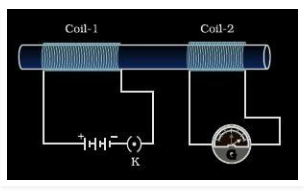From the above two experiments, it was concluded by Faraday that the relative motion between the magnet and the coil resulted in the generation of current in the primary coil. But another experiment conducted by Faraday proved that the relative motion between the coils was not really necessary for the current in the primary to be generated. In this experiment, he placed two stationary coils and connected one of them to the galvanometer and the other to a battery, through a push-button. As the button was pressed, the galvanometer in the other coil showed a deflection, indicating the presence of current in that coil. Also, the deflection in the pointer was temporary and if pressed continuously, the pointer showed no deflection and when the key was released, the deflection occurred in the opposite direction.

Self Inductance for a solenoid

We will take a solenoid having N turns, Let its length be ‘l’ and area of cross-section be ‘A’ where current I is flowing through it. There will be a magnetic field ‘B’ at any given point in the solenoid. Therefore, the magnetic flux per turn will be equal to B × area of each turn.

However, B = (μ0NI)/l

Therefore, magnetic flux per turn = (μ0NIA)/l

Now, the total magnetic flux (φ) that is connected with the solenoid will be given by the product of flux present through every turn and the total number of turns.

Φ = (μ0NIA) x N /l

That is, Φ = (μ0N2IA) /l ….(eq 1)

If L is the self-inductance of the solenoid, then

Φ = LI …..(eq 2)

Combining the equations (1) and (2) from above, we get

L = (μ0N2A) /l

If you have a core that is made up of a magnetic material of permeability μ, then

L = (μN2A) /l